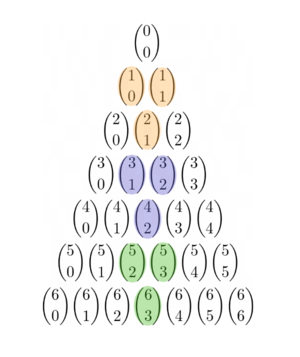# Another way to prove that Pascal's triangle gets larger in the middle?

• Module 3 Week 2 Day 6 Challenge Part 5

Let's look at the 2nd and the 3rd row: 1 1 and 1 2 1.
When you look at the side, the 1's have nothing to add, so they just shift over and are still 1's.
But in the middle, the 1's combine together to form a 2. So 1 2 1.
Now the 3rd and 4th row: 1 2 1 and 1 3 3 1.
The 1's just shifts to the side, but the 2 and the 1's make up the meat: 1 3 3 1.
So Pascal's Triangles is basically where the side 1's shifting overs and the middle numbers getting larger and larger. That explains the mystery on the big middle numbers.

• @RZ923 This is a great explanation.Yes, it's sort of like this: "As the numbers towards the middle get bigger, then the ones even more toward the middle get even bigger..."

This uses the basic rule of Pascal's Triangle: To get any of the numbers in Pascal's Triangle, simply add the two numbers above it. So we get the following:

$$\binom{1}{0} + \binom{1}{1} = \binom{2}{1}$$

$$\binom{3}{1} + \binom{3}{2} = \binom{4}{2}$$

$$\binom{5}{2} + \binom{5}{3} = \binom{6}{3}$$

$$\text{ etc. } \ldots$$That's a heuristic ("hand-wavy") kind of an argument which isn't very rigorous, but also shows why the numbers get bigger as you go towards the middle of Pascal's Triangle!